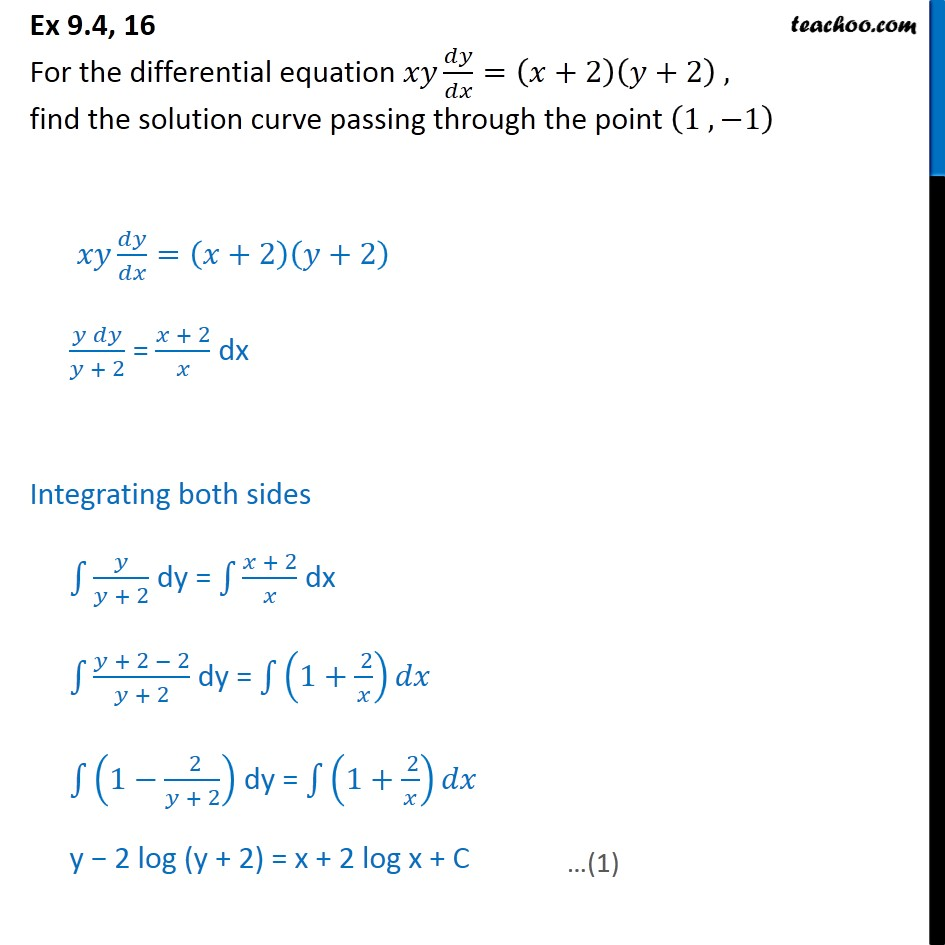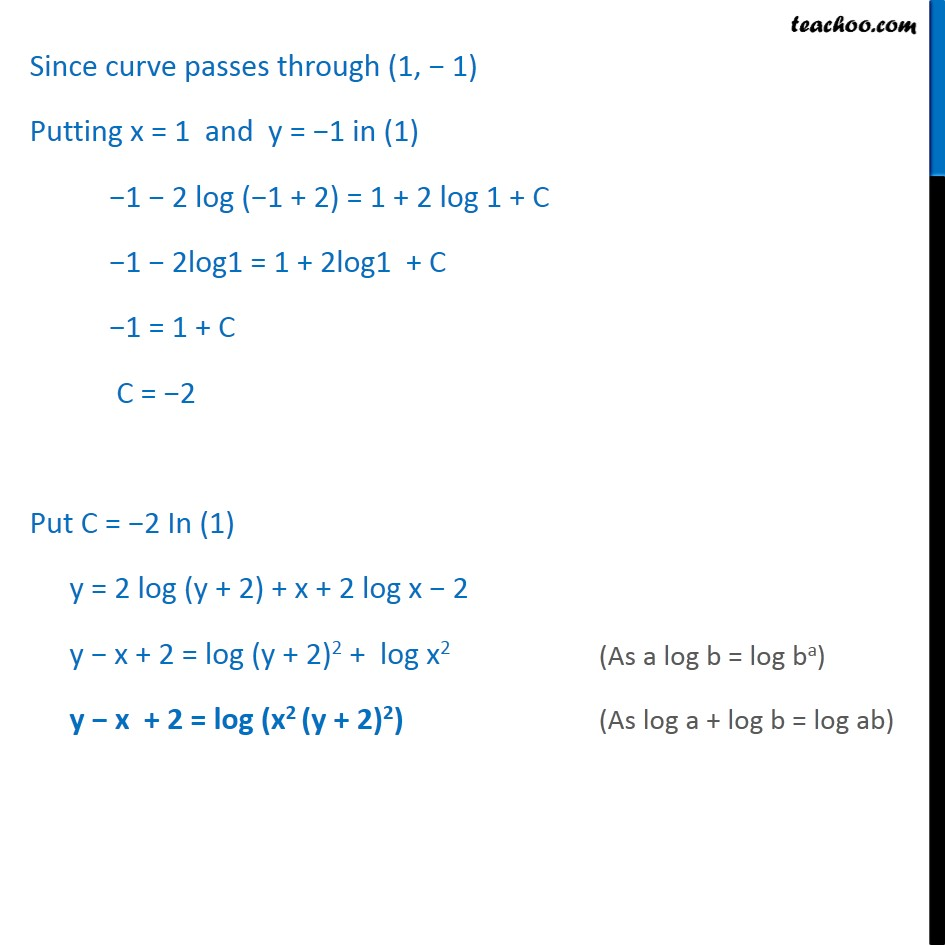Ex 9.3

Chapter 9 Class 12 Differential Equations
Serial order wiseLearn in your speed, with individual attention - Teachoo Maths 1-on-1 Class

### Transcript

Ex 9.3, 16 For the differential equation / =( +2)( +2) , find the solution curve passing through the point (1 , 1) / =( +2)( +2) ( )/( + 2) = ( + 2)/ dx Integrating both sides 1 /( + 2) dy = 1 ( + 2)/ dx 1 ( + 2 2)/( + 2) dy = 1 (1+( 2)/ ) 1 (1 ( 2)/( + 2)) dy = 1 (1+( 2)/ ) y 2 log (y + 2) = x + 2 log x + C Since curve passes through (1, 1) Putting x = 1 and y = 1 in (1) 1 2 log ( 1 + 2) = 1 + 2 log 1 + C 1 2log1 = 1 + 2log1 + C 1 = 1 + C C = 2 Put C = 2 In (1) y = 2 log (y + 2) + x + 2 log x 2 y x + 2 = log (y + 2)2 + log x2 y x + 2 = log (x2 (y + 2)2)